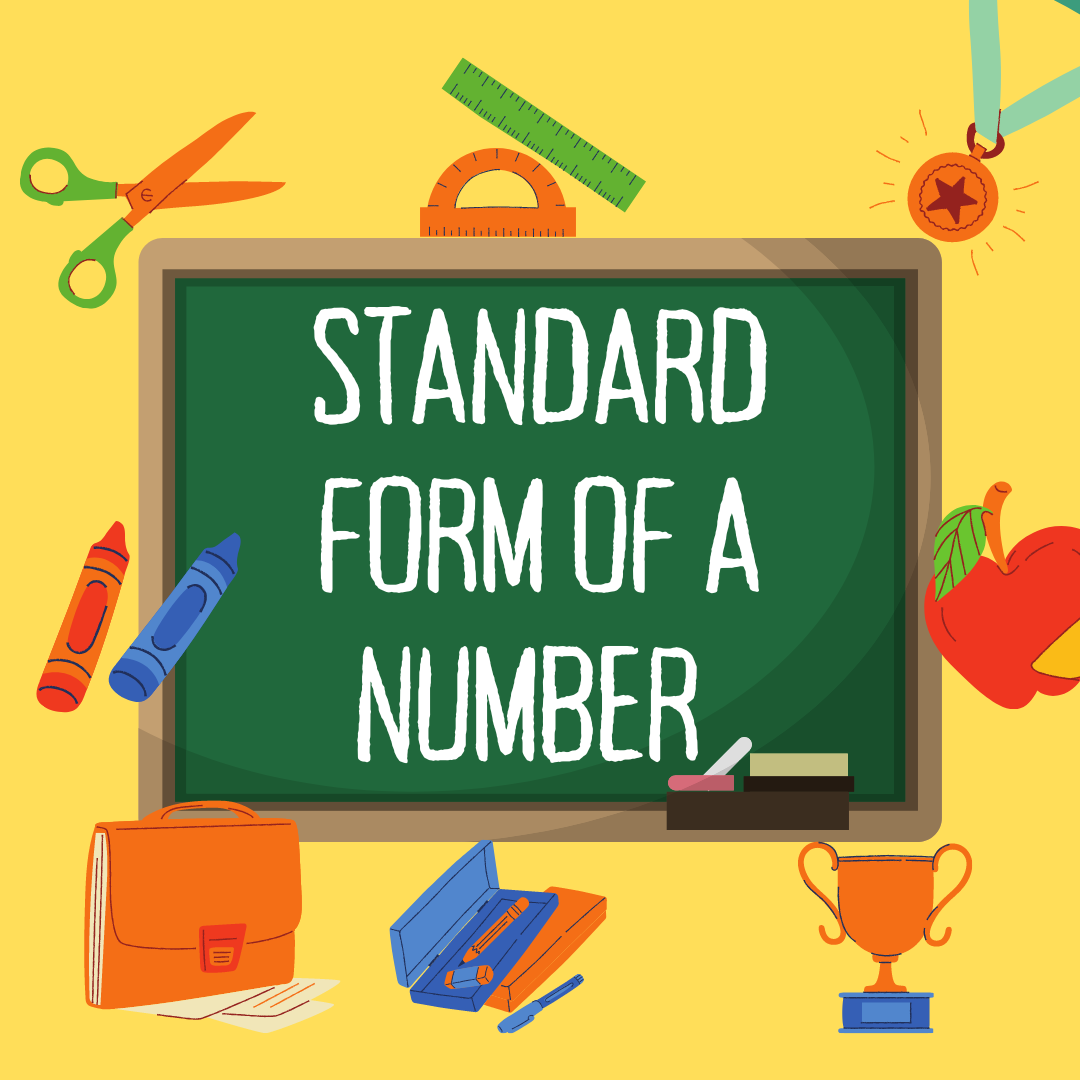# Explanation of Standard Form of a Number with CalculationsIn mathematics, numbers hold significant importance. Numbers can be difficult to read and understand if they have several digits. Mathematicians introduced the standard form of a number to overcome this problem. The standard form is the method of expressing numbers that makes them easier to write and read.

In this blog, we will explore the concept of standard form with its mathematical repression. We will learn how to write fractions, expanded forms, and ordinary numbers into standard forms. In the example section, we will solve some examples for our readers.

## Definition of Standard Form

In mathematics, the standard form is a way of representing numbers in a concise and standardized format. The standard form is also known as scientific notation or exponential notation. Standard form is very helpful when dealing with extremely large or extremely small numbers.

The general format of a number in standard form is a × 10n

Where

• a indicates the decimal number between 1 and 10.
• n represents the exponent or power of 10. The exponent indicates how many positions the decimal point has been moved to the left or right.

The number 5,000,000 can be expressed in standard form as 5 × 106. The number 0.000045 can be written as 4.5 × 10-5. The numbers become more manageable and easier to compare using the standard form.

## Converting Whole Numbers into the Standard Form

You can follow the following steps to convert whole numbers into standard form.

1. Write the first digit from the left of the given whole number.
2. After the first digit, place the decimal point.
3. Count how many numbers of digits after the first digit. This will determine the exponent of 10 in the standard form.

Let's learn these steps by example.

Example: Convert the whole number 3,250,000 to standard form.

Step 1: Write the first digit from the left, which is 3.

Step 2: Put the decimal point after 3.

Step 3: Count how many numbers of digits after the first digit. Here are 6 digits after 3.

Therefore, the standard form 3,250,000 is 3.25 × 106.

A standard form calculator is an online tool that is used to convert numbers in standard notation according to the above-mentioned steps.

## Representing Decimal Numbers into Standard Form

Follow these steps to write a decimal number in standard form.

1. Write the first nonzero digit of the given number.
2. Place the decimal point after it.
3. Determine the number of times the decimal point needs to move to obtain the original number.
4. Write a negative sign on the exponent of 10 if the decimal point moves to the right.

Example: let's convert the decimal number 0.0000000000893 in standard form.

Step 1: The first non-zero digit is 5.

Step 2: Place the decimal point after 5.

Step 3: The decimal point moves 11 places to the right to obtain the original number.

Step 4: Since the decimal point moves to the right, we write a negative sign on the exponent of 10.

Thus, the standard form of 0.0000000000893 is 8.93 × 1011.

## Writing Expanded form as Standard form

Steps are given in the following to write the expanded form in standard form.

1. Identify the given number in expanded form.

Example: 4 × 105 + 2 × 103 + 7 × 10-2

1. To simplify the expression, perform the arithmetic operations in the expanded form.

Example: 4 × 100000 + 2 × 1000 + 7 × 0.01

1. Calculate the values in the expanded form.

Example: 400000 + 2000 + 0.07

1. Add up all the values to obtain the result.

Example: 402000.07

## Method to write the rational number (Fraction) in standard form

A rational number p/q is expressed in its standard form when

• The denominator (q) is positive
• The numerator p and denominator q have no common factors other than 1.

To convert a given rational number into its standard form, follow these steps

1. Write down the given rational number.
2. The numerator and denominator will be multiplied by -1 if the denominator is negative.
3. Determine the greatest common divisor of the p and q.
4. Cancel out the GCD from the numerator and the denominator. This step ensures that p and q have no common divisors other than 1.
5. The resulting fraction will be the rational number's standard form.

Example: Write the standard form of the rational number 14/21.

Step 1: Identify the given rational number 14/21.

Step 2: The GCD of 14 and 21 is 7.

Step 3: Divide the numerator and the denominator by 7.

(14 ÷ 7) / (21 ÷ 7) = 2/3

Thus, the standard form of 14/21 is 2/3.

## Solved Examples of Standard Form and Scientific notation

Let's go through some examples of standard form and scientific notation.

Example 1:

Represent the distance from the Earth to the Sun in scientific notation.

Solution:

The distance from the Earth to the Sun is about 93000000 miles.

Thus,

93,000,000 miles = 9.3 × 107 miles

Example 2:

Write the mass of an electron in standard form.

Solution:

The mass of an electron is approximately 0.00000000000000000000000000000091 kilograms

0.00000000000000000000000000000091 kg = 9.1 × 10-31 kilograms

Hence, the mass of an electron in standard form is 9.1 × 10-31 kilograms

## Conclusion

In this article, we examined the standard form in detail. We learned how to write any form of a number in its standard form. Different solved examples have been provided for you for mastering the standard form. After reading this article, you will be able to represent any number in standard form and understand its significance.